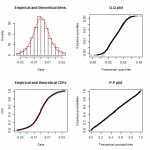# Generalized linear functions (Beginners)

September 16, 2017
By

Want to share your content on R-bloggers? click here if you have a blog, or here if you don't.On this set of exercises, we are going to use the `lm` and `glm` functions to perform several generalized linear models on one dataset.

Since this is a basic set of exercises we will take a closer look at the arguments of these functions and how to take advantage of the output of each function so we can find a model that fits our data.

Before starting this set of exercises, I strongly suggest you look at the R Documentation of `lm` and `glm`.

Note: This set of exercises assume that you have a basic understanding of generalized linear models.

Answers to the exercises are available here.

If you obtained a different (correct) answer than those listed on the solutions page, please feel free to post your answer as a comment on that page.

The dataset we will be using contains information from passengers of the Titanic including if they survived or not.

To obtain the data run these lines of code.

``` if (!'titanic' %in% installed.packages()) install.packages('titanic') library(titanic) DATA <- titanic_train[,-c(1,4,9,11)] ```

Exercise 1

Linear regression

1. Use `DATA` to create a linear model using the function `lm` with the variables Age and Fare as independent variables and Survived as the independent one. Save the regression in an object called `lm_reg`

2. Use the function `glm` to perform the same task and save the regression in an object called `glm_reg`

Exercise 2

If you print any of the previous objects you will realize that there’s not much information about the performance of the models, fortunately `summary` is a great function to find out more about any statistical model you preform to a dataset. Depending on the model `summary` will produce different outputs.

• Apply `summary` to `lm_reg` and to `glm_reg`. You will find a slight difference between both of the outputs, that is because `glm` is more flexible than `lm`.

Exercise 3

So far we have been assuming (incorrectly) that the dependent variable (`Survived`) follows a normal distribution and that’s why we have been performing a linear regression. Obviously `Survived` follows a binomial distribution, there are only two options either the passenger survived (1) or the passenger wasn’t that lucky and he died (0). Since the data has a binomial distribution we should perform a logistic regression, to do this use the function `glm` to perform a logistic regression using `Age` and `Fare` as independent variables and save it in an object called `bin_model`. Hint: Define the value of the argument `family` properly.

Exercise 4

Inside the family attribute you can always specify a particular link, in case you don’t a default link will be associated depending on the family you chose.

1. To find out the default link associated to a certain family, you can write the family name followed by a parenthesis (Ex. `gaussian()`. Find the default link associated to the binomial family.
2. Create a probit model with the same variables used in `bin_model` and save it in an object called `bin_probit_model`.

Exercise 5

Findind the right model requires to compare different models and choose the best, although there are many performance measures, for now we will use the `AIC` as our measure (smaller AIC are better). This means that `bin_model` is better than `bin_probit_model`, so let’s keep working with `bin_model`.

Until now intercept variable has been part of the models. Create a logistic regression with the same variables but with no intercept.

Exercise 6

Impute data. If you run the `summary` function to any of the previous models you will find out that 177 observations have been deleted due to missingness. This happens because the `glm` function has as default argument `na.acton ="na.omit"`. This make easier to run a model with messier data, but that is not always great. You want to have full control an understanding of what does the function is doing.

1. There are some missing values in `age`, replace this values with the median.
2. Update the `glm_model` with the updated data, specify `na.action='na.fail'` This will assure us that the dataset has no missing values, otherwise it will show an error.

Learn more about evaluating different statistical models in the online courses Linear regression in R for Data Scientists and Structural equation modeling (SEM) with lavaan. These courses cover different statistical models that can help you choose the right design for your solution.

Exercise 7

Add polynomial independent variables. Some variables have a quadratic interaction between them and the dependent variable, this can be solved by specifying in the formula of the model a quadratic interaction.

Add a quadratic interaction for the variable `Fare` into the current model, specified in `glm_model`

Exercise 8

Add categorical variables.  Add `Sex` as an independent variable  into the current model specified in `glm_model`. Note that Sex is not a numeric variable.

Exercise 9

Now that we have found a good model that fits our data, so it’s time to use the `predict` function to find how good the model predicts in our own data. Use the function `predict` to find the prediction of the model in `DATA` and save it in `Pred.default`

Exercise 10

`Pred.default` shows the predicted values under the link transformation, in this case logit. This is not easily interpretable, to fix this problem we can specify the `type` of prediction we want.

Obtain the predictions as probability values.
Exta: What’s the percentage accuracy of this model if we assigned as died (0) if the predicted probability is less than 0.5 and survived (1) otherwise?

R-bloggers.com offers daily e-mail updates about R news and tutorials about learning R and many other topics. Click here if you're looking to post or find an R/data-science job.
Want to share your content on R-bloggers? click here if you have a blog, or here if you don't.